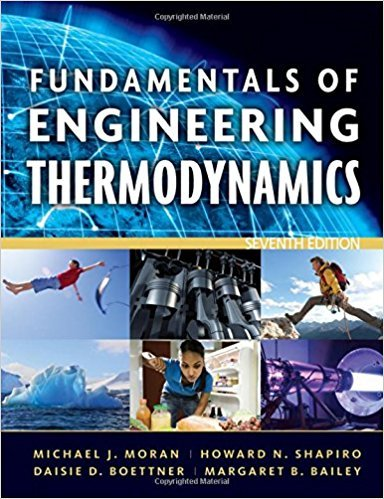×
×

# Carnot Cycle ApplicationsOne-tenth kilogram of air as anISBN: 9780470495902 50

## Solution for problem 82P Chapter 5

Fundamentals of Engineering Thermodynamics | 7th Edition

• Textbook Solutions
• 2901 Step-by-step solutions solved by professors and subject experts
• Get 24/7 help from StudySoup virtual teaching assistantsFundamentals of Engineering Thermodynamics | 7th Edition

4 5 1 426 Reviews
16
3
Problem 82P

Carnot Cycle Applications

One-tenth kilogram of air as an ideal gas with k = 1.4 executes a Carnot refrigeration cycle, as shown in Fig. 5.16. The isothermal expansion occurs at −23°C with a heat transfer to the air of 3.4 kJ. The isothermal compression occurs at 27°C to a final volume of 0.01 m3. Using the results of Prob. 5.80 adapted to the present case, determine

(a) the pressure, in kPa, at each of the four principal states.

(b) the work, in kJ, for each of the four processes.

(c) the coefficient of performance.

Prob. 5.80

Carnot Cycle Applications

The pressure-volume diagram of a Carnot power cycle executed by an ideal gas with constant specific heat ratio k is shown in Fig. P5.80. Demonstrate that

(a) V4V2 = V1V3.

(b) T2/T3 = (p2/p3)(k-1)/k.

(c) T2/T3 = (V3/V2)(k-1).Fig. P5.80Fig. 5.16 p?v diagram for a Carnot gas refrigeration or heat pump cycle.

Step-by-Step Solution:

Step 1 of 6</p>

Considerof air executes a carnot refrigeration cycle in which the isothermal expansion occurs atwith a heat transfer to the air ofand the isothermal compression occurs atto a final volume of.

We need to find out the pressure at each of the four principal states, the work for each of the four processes and the coefficient of performance.

Step 2 of 6</p>

We have,And,a) Using the ideal gas equation,For process,Step 3 of 6</p>

The volume atis,For processSO,So,Thus,Step 4 of 5

Step 5 of 5

##### ISBN: 9780470495902

This full solution covers the following key subjects: cycle, Carnot, fig, heat, gas. This expansive textbook survival guide covers 14 chapters, and 1501 solutions. Since the solution to 82P from 5 chapter was answered, more than 1099 students have viewed the full step-by-step answer. The full step-by-step solution to problem: 82P from chapter: 5 was answered by , our top Engineering and Tech solution expert on 07/20/17, 09:01AM. Fundamentals of Engineering Thermodynamics was written by and is associated to the ISBN: 9780470495902. This textbook survival guide was created for the textbook: Fundamentals of Engineering Thermodynamics, edition: 7. The answer to “Carnot Cycle ApplicationsOne-tenth kilogram of air as an ideal gas with k = 1.4 executes a Carnot refrigeration cycle, as shown in Fig. 5.16. The isothermal expansion occurs at ?23°C with a heat transfer to the air of 3.4 kJ. The isothermal compression occurs at 27°C to a final volume of 0.01 m3. Using the results of Prob. 5.80 adapted to the present case, determine(a) the pressure, in kPa, at each of the four principal states.________________(b) the work, in kJ, for each of the four processes.________________(c) the coefficient of performance.Prob. 5.80Carnot Cycle ApplicationsThe pressure-volume diagram of a Carnot power cycle executed by an ideal gas with constant specific heat ratio k is shown in Fig. P5.80. Demonstrate that(a) V4V2 = V1V3.(b) T2/T3 = (p2/p3)(k-1)/k.(c) T2/T3 = (V3/V2)(k-1). Fig. P5.80 Fig. 5.16 p?v diagram for a Carnot gas refrigeration or heat pump cycle.” is broken down into a number of easy to follow steps, and 142 words.

Unlock Textbook Solution